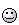# Calculation of Light Intensity

Hi all,

I would like to calculate light intensity of a bulb from 0.5 meter. Which formula shoul i use? I have a PV. Dimensions are 12x12 cm. Bulb is 100 Watt.

Thanks

The actual amount of visible light that a 100W bulb emits will depend on the type of bulb and, particularly with a filament bulb, the temperature it runs at. So the bottom line is that you need more information about the bulb before you can tell.
If you are using a PV panel, you also need to know the spec. Most PV panels are specified for use in Sunlight, too, so the simple information that would be published about the panel would probably not be enough.
The easiest way to find out what you want to know would be to do an experiment - but that would involve actually buying a PV panel and a 100W bulb, which may not appeal to you.The package for the light bulb should state the number of lumens the bulb makes when operated at the rated voltage. Use of a PV panel is irrelevant as long as it is adequate for the power and voltage required. (Be careful, most PV panels make 20V DC, while most bulbs need 120V or 230V AC)

Lookup the unit of light lumen.

I would like to calculate light intensity of a bulb from 0.5 meter. Which formula shoul i use? I have a PV. Dimensions are 12x12 cm. Bulb is 100 Watt.
Reading "between the lines", I suspect that in the end, you want to calculate how much power (in watts) the PV panel will produce.

If so, this is not an easy calculation. First, you need to know not only how much electromagnetic power the bulb radiates, but also its spectrum: how it is distributed among different wavelengths. This varies from one kind of bulb to another, as sophiecentaur noted. Also, you need to know how the panel's sensitivity varies with wavelength. As sophiecentaur noted, if the panel's specifications indicate a certain number of watts, it probably assumes sunlight, which has a spectrum that probably doesn't match your bulb. Finally, you need to know geometrically, how much of the radiation emitted by the panel is intercepted by the panel, which has to do with the solid angle of the panel relative to the bulb.

The package for the light bulb should state the number of lumens the bulb makes when operated at the rated voltage.

Lumens don't tell you the electromagnetic power radiated by the bulb. They're a measure of the brightness as perceived by the human eye, that is, they're calculated according to the sensitivity of the eye as a function of wavelength.

•Klystron and davenn
Lumens don't tell you the electromagnetic power radiated by the bulb. They're a measure of the brightness as perceived by the human eye, that is, they're calculated according to the sensitivity of the eye as a function of wavelength.

I suspect the perception is what the OP wants.

Perhaps @tornado would tell us what this is all about - I had perceived it entirely differently - the 100W bulb illuminating a PV panel, which would produce some electrical power.
The other way round is equally likely though.

•anorlunda
Perhaps @tornado would tell us what this is all about - I had perceived it entirely differently - the 100W bulb illuminating a PV panel, which would produce some electrical power.
The other way round is equally likely though.Hah! I never thought of it that way. I guess we really do need @tornado to clarify.

There are many ways to approach this. If you want a first principles argument, then assume the bulb acts as a perfect Planckian emitter. You need to estimate the area of the filament and then use the Stefan-Boltzmann equation to calculate the temperature to give you 100W of optical flux. From the temperature you can calculate the spectral power distribution using Planck's Law. Multiplying that by the photopic response curve gives you lumens.

A shortcut would be to determine the LER (luminous efficacy of radiation) for a given temperature. For a black body radiator efficacy from 2000 - 3200 K is given by 1.3051e-5 T^2 - 4.4624e-2 T + 3.8696e+1 (T = [K]). A typical incandescent will be between about 2800 and 3000 K. 100W x LER gives luminous flux (lumens). The temperature still let's you calculate the spectral power distribution via Planck's Law if you are concerned about wavelength efficiency for a PV cell.

You can also use the Energy Star equivalents for LED replacement bulbs to estimate luminous flux. https://www.energystar.gov/sites/default/files/ENERGY STAR Lamps V2.1 Final Specification.pdf

(Be careful, most PV panels make 20V DC, while most bulbs need 120V or 230V AC)
1. I read it as illuminating the PV panel with the bulb to obtain a measure of its intensity, not using the PV panel to power the bulb.

2. A normal filament bulb will run equally well from DC as it will on AC. The AC voltage rating is the RMS value, which is equivalent to the same value in DC.

Last edited by a moderator:
It appears that the OP has not returned here since posting to read any of the replies.